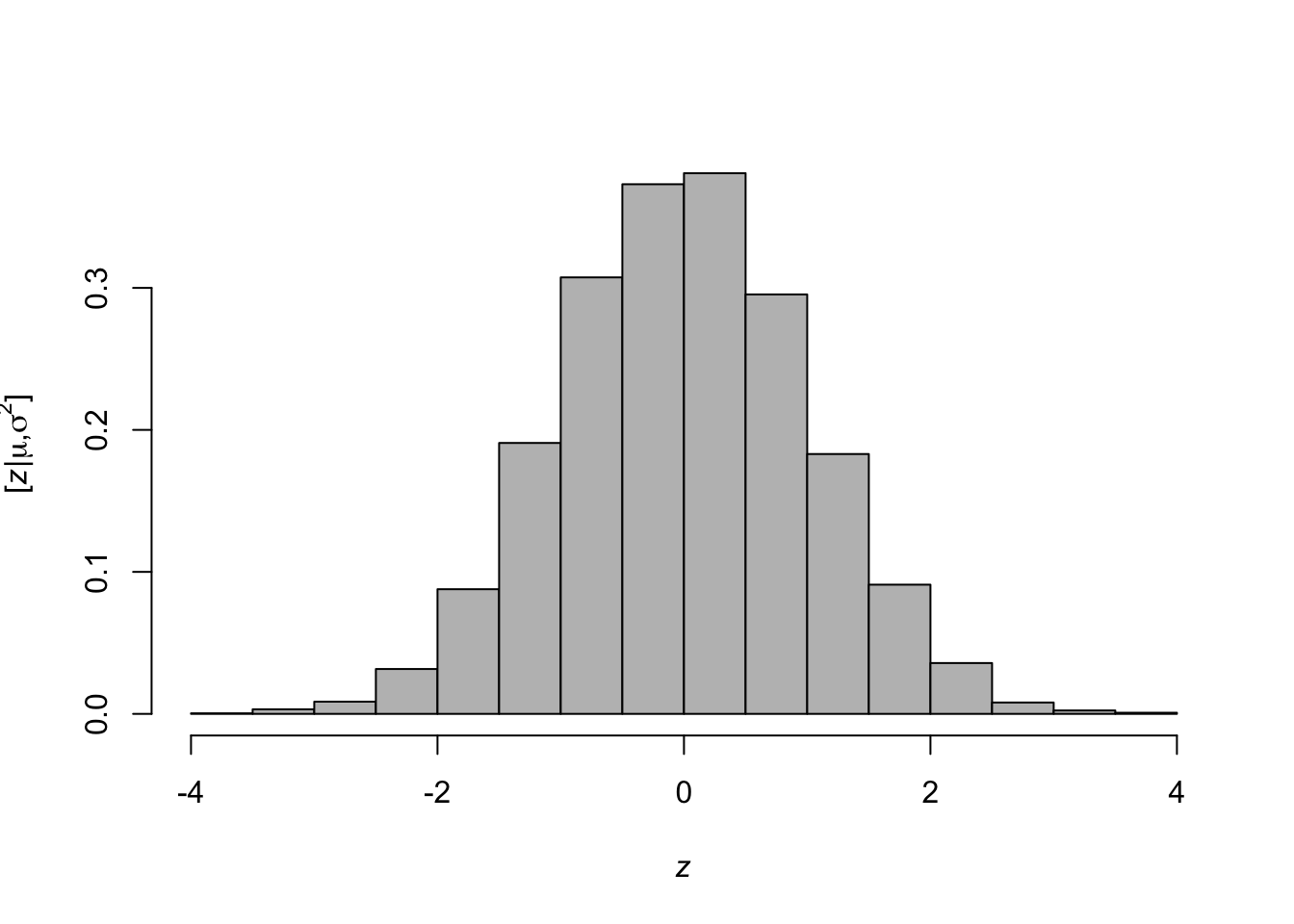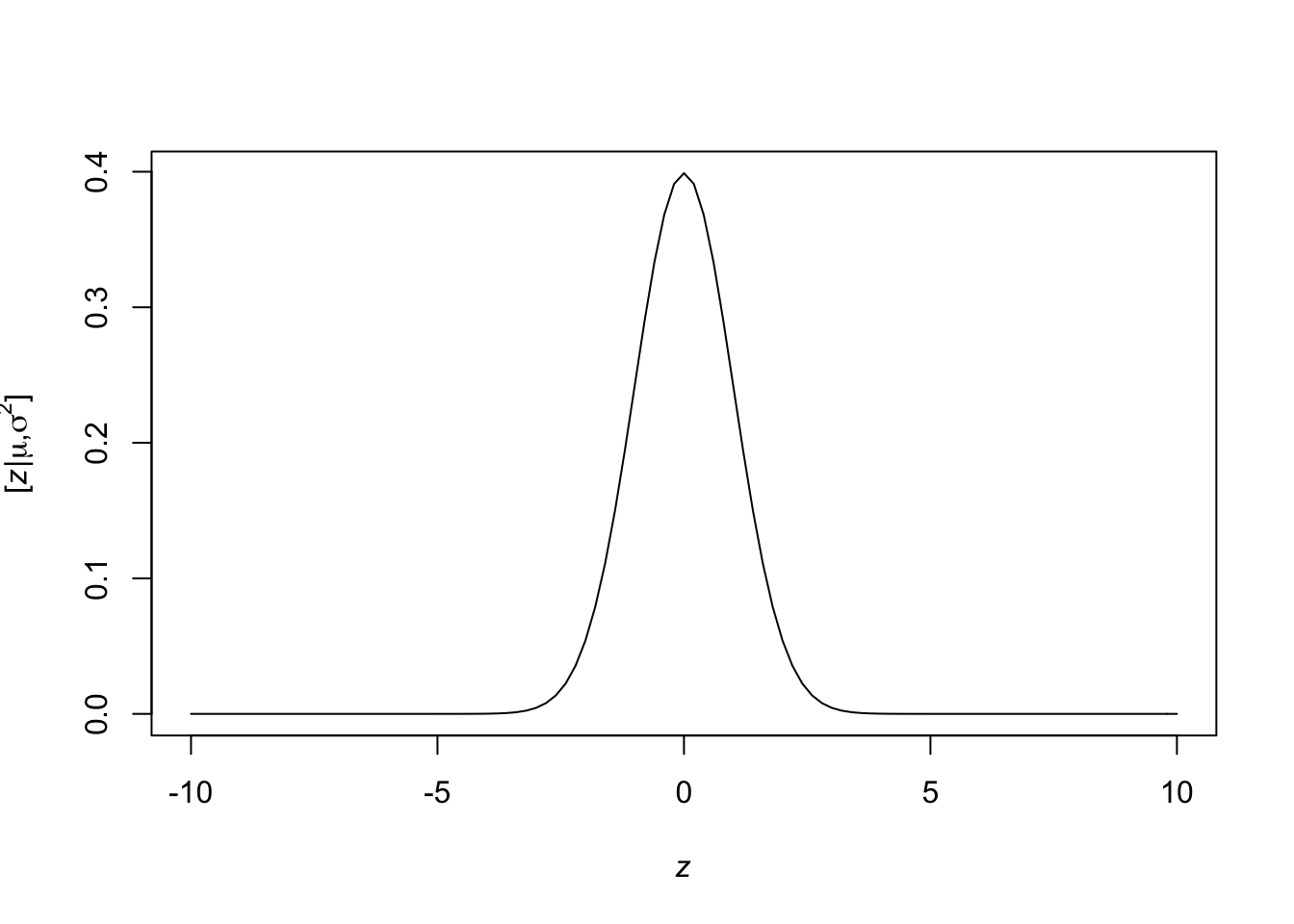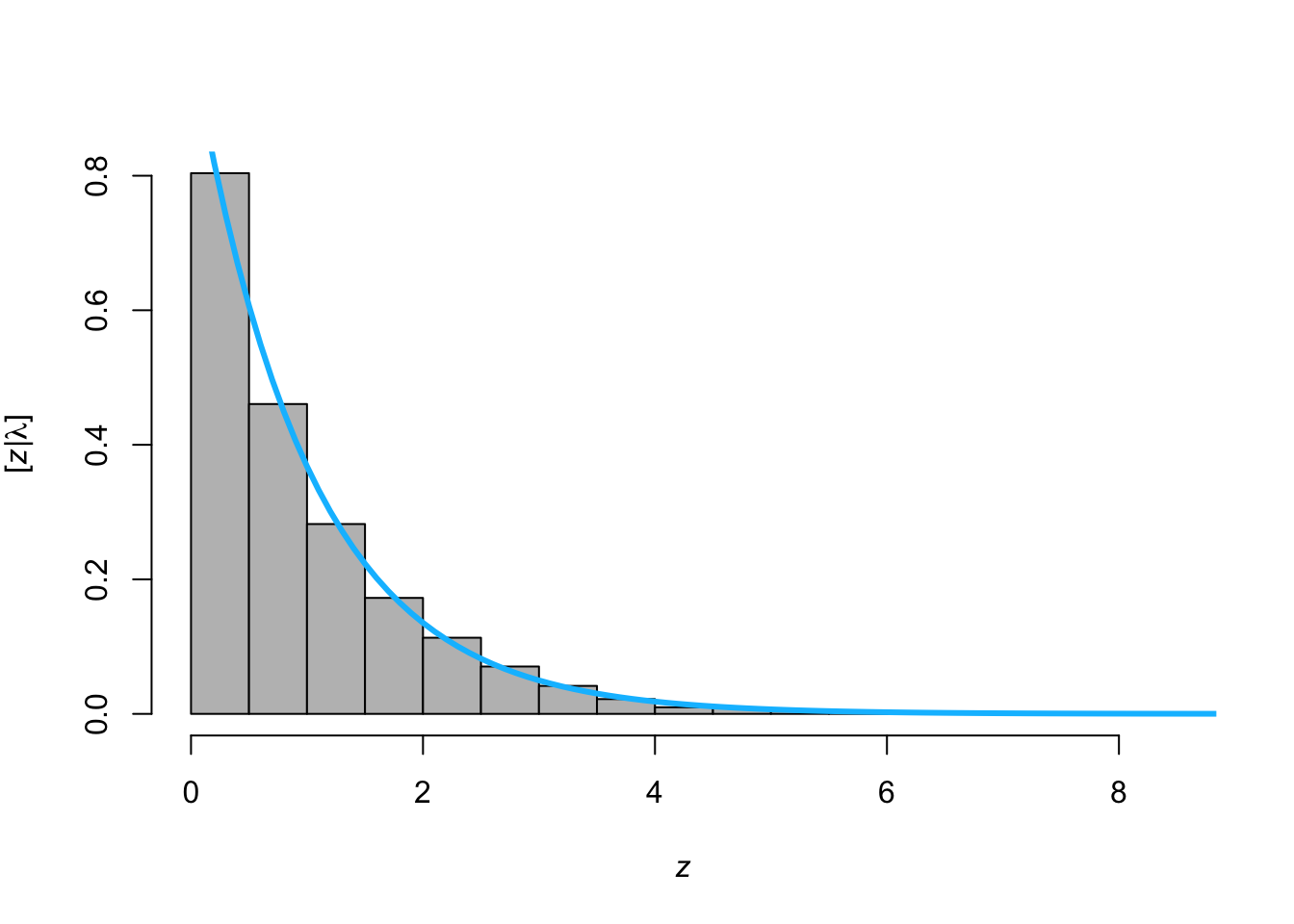# 2 August 20

## 2.1 Announcements

• Assignment #1 due Friday at 5 pm
• Office hours 9:30 - 10:30 today

## 2.2 Statistical models

• What is a model?
• Simplification of something that is real designed to serve a purpose
• What is a statistical model?
• Simplification of a real data generating mechanism
• Constructed from deterministic mathematical equations and Probability density /mass functions
• Capable of generating data
• What is the purpose of a statistical model
• See section 1.2 on pg. 7 and pg. 77 of Wikle et al. (2019)
• Capable of making predictions, forecasts, and hindcasts
• Enables statistical inference about observable and unobservable quantities
• Reliability quantify and communicate uncertainty
• Example using simple linear regression

## 2.3 Matrix review

• Column vectors
• $$\mathbf{y}\equiv(y_{1},y_{2},\ldots,y_{n})^{'}$$
• $$\mathbf{x}\equiv(x_{1},x_{2},\ldots,x_{n})^{'}$$
• $$\boldsymbol{\beta}\equiv(\beta_{1},\beta_{2},\ldots,\beta_{p})^{'}$$
• $$\boldsymbol{1}\equiv(1,1,\ldots,1)^{'}$$
• In R
y <- matrix(c(1,2,3),nrow=3,ncol=1)
y
##      [,1]
## [1,]    1
## [2,]    2
## [3,]    3
• Matrices
• $$\mathbf{X}\equiv(\mathbf{x}_{1},\mathbf{x}_{2},\ldots,\mathbf{x}_{p})$$
• In R
X <- matrix(c(1,2,3,4,5,6),nrow=3,ncol=2,byrow=FALSE)
X
##      [,1] [,2]
## [1,]    1    4
## [2,]    2    5
## [3,]    3    6
• Vector multiplication
• $$\mathbf{y}^{'}\mathbf{y}$$
• $$\mathbf{1}^{'}\mathbf{1}$$
• $$\mathbf{1}\mathbf{1}^{'}$$
• In R
t(y)%*%y    
##      [,1]
## [1,]   14
• Matrix by vector multiplication
• $$\mathbf{X}^{'}\mathbf{y}$$
• In R
t(X)%*%y
##      [,1]
## [1,]   14
## [2,]   32
• Matrix by matrix multiplication
• $$\mathbf{X}^{'}\mathbf{X}$$
• In R
t(X)%*%X
##      [,1] [,2]
## [1,]   14   32
## [2,]   32   77
• Matrix inversion
• $$(\mathbf{X}^{'}\mathbf{X})^{-1}$$
• In R
solve(t(X)%*%X)
##            [,1]       [,2]
## [1,]  1.4259259 -0.5925926
## [2,] -0.5925926  0.2592593
• Determinant of a matrix
• $$|\mathbf{I}|$$
• In R
I <- diag(1,3)
I
##      [,1] [,2] [,3]
## [1,]    1    0    0
## [2,]    0    1    0
## [3,]    0    0    1
det(I)
##  1
• $$\mathbf{y}^{'}\mathbf{S}\mathbf{y}$$
• Derivative of a quadratic form (Note $$\mathbf{S}$$ is a symmetric matrix; e.g., $$\mathbf{X}^{'}\mathbf{X}$$)
• $$\frac{\partial}{\partial\mathbf{y}}\mathbf{y^{'}\mathbf{S}\mathbf{y}}=2\mathbf{S}\mathbf{y}$$
• Other useful derivatives
• $$\frac{\partial}{\partial\mathbf{y}}\mathbf{\mathbf{x^{'}}\mathbf{y}}=\mathbf{x}$$
• $$\frac{\partial}{\partial\mathbf{y}}\mathbf{\mathbf{X^{'}}\mathbf{y}}=\mathbf{X}$$

## 2.4 Distribution theory review

• Probability density functions (PDF) and probability mass functions (PMF)
• Normal distribution (continuous support)
• Binomial distribution (discrete support)
• Poisson distribution (discrete support)
• And many more (see handout)
• Distributions in R
• PDF of the normal distribution $[z|\mu,\sigma^2] = \frac{1}{\sqrt{2\pi\sigma^2}}\textit{e}^{-\frac{1}{2\sigma^2}(z - \mu)^2}$
• $$z$$ is the random variable
• $$\mu$$ and $$\sigma^2$$ are the parameters
• PDFs & PMFs in R
• ?dnorm
• Generate random variables ($$z$$) from a PDF (e.g., $$z_i\sim\text{N}(\mu,\sigma^2)$$)
z <- rnorm(n = 5, mean = 0, sd = 1)
z    
##  -0.7393080  0.7771272 -0.3673657  1.0109616  1.0145325
• Histogram representation of a PDF
library(latex2exp)
z <- rnorm(n = 10000, mean = 0, sd = 1)
hist(z,freq=FALSE,col="grey",main = "",
xlab= TeX('$\\mathit{z}$'),
ylab = TeX('$\\lbrack\\mathit{z}|\\mu,\\sigma^2\\rbrack$'))• Plot a PDF in R
curve(expr = dnorm(x = x, mean = 0, sd = 1), from = -10, to = 10,
xlab= TeX('$\\mathit{z}$'),
ylab = TeX('$\\lbrack\\mathit{z}|\\mu,\\sigma^2\\rbrack$'))• Evaluate the “likelihood” at a given value of the parameters
dnorm(x = 1.759, mean = 0, sd = 1, log = FALSE)
##  0.08492566
• Other distributions
rpois(n = 5, lambda = 2)
rbinom(n = 5, size = 10, prob = 0.5)
runif(n = 5,min = 0,max = 3)
rt(n = 5,df = 1)
rcauchy(n = 5, location = 2, scale = 4)
• Making your functions for a distribution
• PDF of the exponential distribution $[z|\lambda] = \lambda\textit{e}^{-\lambda z}$
• Make your own function for the PDF of the exponential distribution
dexp <- function(z, lambda){lambda*exp(-lambda*z)}
• Make your own function to simulate random variables from the exponential distribution using the inverse probability integral transform
rexp <- function(n, lambda){
u <- runif(n)
-1/lambda*log(1-u)
}
• Make histogram by sampling from rexp() and overlay the PDF using dexp()
z <- rexp(n = 10000, lambda = 1)
hist(z,freq=FALSE,col="grey",main = "",
xlab= TeX('$\\mathit{z}$'),
ylab = TeX('$\\lbrack\\mathit{z}|\\lambda\\rbrack$'))
curve(expr = dexp(z = x,lambda = 1), from = 0, to = 10,
add = TRUE,col = "deepskyblue",lwd = 3)• Moments of a distribution
• First moment: $$\text{E}(z) = \int z [z|\theta]dz$$
• Second central moment: $$\text{Var}(z) = \int (z -\text{E}(z))^2[z|\theta]dz$$
• Note that $$[z|\theta]$$ is an arbitrary PDF or PMF with parameters $$\theta$$
• Example normal distribution $\begin{eqnarray} \text{E}(z) &=& \int_{-\infty}^\infty z\frac{1}{\sqrt{2\pi\sigma^2}}\textit{e}^{-\frac{1}{2\sigma^2}(z - \mu)^2}dz\\&=& \mu \end{eqnarray}$ $\begin{eqnarray} \text{Var}(z) &=& \int_{-\infty}^\infty (z-\mu)^2\frac{1}{\sqrt{2\pi\sigma^2}}\textit{e}^{-\frac{1}{2\sigma^2}(z - \mu)^2}dz\\&=& \sigma^2 \end{eqnarray}$
• Example exponential distribution$\begin{eqnarray} \text{E}(z) &=& \int_{0}^\infty z\lambda\textit{e}^{-\lambda z}dz\\&=& \frac{1}{\lambda} \end{eqnarray}$$\begin{eqnarray}\text{Var}(z) &=& \int_{0}^\infty (z-\mu)^2\lambda\textit{e}^{-\lambda z}dz\\&=& \frac{1}{\lambda^2} \end{eqnarray}$

## 2.5 Mathematical model review

• Mathematical models are deterministic equations that describe the relationship between input variables and an output variable
• Common types of mathematical models used for spatio-temporal statistics
• Linear equations
• Scalar form: $$\mu=\beta_{0}+\beta_{1}x_{1}+\beta_{2}x_{2}+\ldots+\beta_{p}x_{p}$$
• Vector form: $$\boldsymbol{\mu}=\beta_{0}+\beta_{1}\mathbf{x}_{1}+\beta_{2}\mathbf{x}_{2}+\ldots+\beta_{p}\mathbf{x}_{p}$$
• Matrix form: $$\boldsymbol{\mu}=\mathbf{X}\boldsymbol{\beta}$$
• Non-linear equations Scalar form: $$\mu = e^{\beta_{0}+\beta_{1}x_{1}+\beta_{2}x_{2}+\ldots+\beta_{p}x_{p}}$$
• Difference equations
• Scalar form: $$\mu_{t+1} = \phi\mu_{t}$$
• Differential equations
• Scalar form: $$\frac{d\mu(t)}{dt}=\gamma\mu(t)$$